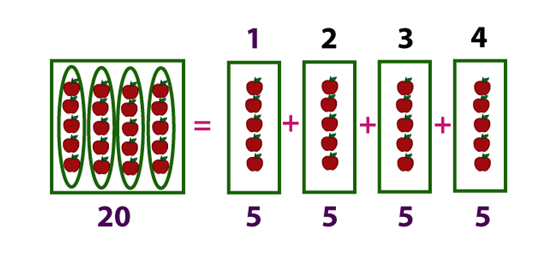# Division Formula

There are four basic operations of Arithmetic, namely, Addition, Subtraction, Multiplication and Division.

Division is breaking a number into an equal number of parts. Division is an arithmetic operation used in Maths. It splits a given number of items into different groups.

For instance, 20 divided by 4: If you take 20 apples and put them into four equal-sized groups, there will be 5 apples in each group.Division Sign

There are a number of signs that people may use to indicate division. The most common one is ÷, but the backslash / and a horizontal line (-) is also used in the form of Fraction, where a Numerator is written on the top and Denominator on the bottom.

Example signs for “a divided by b”:

$$\begin{array}{l}a÷b\end{array}$$

$$\begin{array}{l}\frac{a}{b}\end{array}$$

$$\begin{array}{l}a/b\end{array}$$

The Division formula is –

$\LARGE Dividend \div Divisor = Quotient$

OR

$\LARGE \frac{Dividend}{Divisor} = Quotient$

Where,
Dividend is the number to be divided
Divisor is the number to be divided with
Quotient is the result to be found after division

Special Cases

During the Division operation, there are three special cases to consider,

• Dividing by 1: When any number is divided by 1, the answer remains the same. In other words, if the divisor is 1 then the quotient equals the dividend.

Examples: 40 ÷ 1 = 40

2.5 ÷ 1 = 2.5

• Dividing by 0: A number cannot be divided 0. The answer to this question is undefined.
• Dividend equals Divisor: If the dividend and the divisor are the same numbers (not 0), then the answer is always 1.

Examples: 40 ÷ 40 = 1

2.5 ÷ 2.5 = 1

### Solved Example

Question: Solve: 221 ÷ 13

Solution:

13) 221 (17
13
————-
91
91
————-
00
$$\begin{array}{l}Therefore,\end{array}$$
$$\begin{array}{l}221 ÷ 13 = 17\end{array}$$